# Civil Engineering - Strength of Materials - Discussion

### Discussion :: Strength of Materials - Section 5 (Q.No.23)

23.

If p is the internal pressure in a thin cylinder of diameter d and thickness t, the developed hoop stress, is

 [A].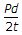[B].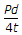[C].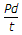[D].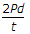[E].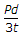Explanation:

No answer description available for this question.

 Jinish said: (Aug 16, 2019) Hoop stresses is perpendicular to both axis and radius of the cylinder and calculated by pd/2t formula and longitudinal or axial stress can be found out by pd/4t formula in the thin cylinder. Where p is internal pressure and t is the thickness of cylinder and thickness is 1/20 of the cylinder diameter and d is length of the cylinder.